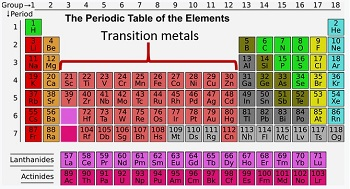# Using Ammonium Hydroxide & Sodium Hydroxide on Metals & Metal Oxides

Instructor: Matthew Bergstresser

Matthew has a Master of Arts degree in Physics Education. He has taught high school chemistry and physics for 14 years.

Ammonium hydroxide and sodium hydroxide are bases. They react with metals and metal oxides forming metal hydroxides. In this lesson, we will investigate these reactions.

## Acids and Bases

Do you know anyone who can write with both hands? People that have that capability are called ambidextrous. This is comparable to how certain chemical substances can act as an acid or a base. Metals generally react with acids and metallic oxides are usually basic and react with acids also. Certain metals act with acids and bases. As we move from the left side of the periodic table where the alkali metals and alkaline metals are located towards the right side of the periodic table we go through the transition metals. Several transition metal oxides are amphoteric, which means they react with acids and bases. Let's focus on two of the amphoteric substances and how they react with bases.## Zinc

### Reaction with Ammonium Hydroxide

Zinc metal (Zn) reacts with ammonium hydroxide, which is a weak base. Because of this, ammonium hydroxide is written as ammonia (NH3) plus water. Let's look at the reaction.

Zn+2 (aq) + 2NH3 (aq) + 2H2 O (l) → Zn(OH)2 (s) + 2NH4 + (aq)

The zinc ion (Zn+2) accepts the pair of electrons

What is unusual about this reaction is the solid zinc hydroxide dissolves if more ammonia is added. In other words, zinc reacts with ammonia to form zinc hydroxide and upon the addition of extra ammonia, the zinc hydroxide dissolves. Let's see what happens when zinc reacts with sodium hydroxide.

### Reaction with Sodium Hydroxide

Zinc reacts with the strong base sodium hydroxide (NaOH) according to the equation

Zn (s) + 2H2 O (l) + 2NaOH (aq) → Na2 Zn(OH)4 (s) + H2 (g)

The sodium and hydroxide completely separate, which makes sodium hydroxide a strong base. Notice the zinc is not replacing the sodium because sodium is more reactive. Zinc is replacing hydrogen. The same reaction occurs when zinc oxide (ZnO) reacts with sodium hydroxide.

Let's now look at another amphoteric substance, which involves copper.

## Copper

### Reaction with Ammonium Hydroxide

Copper is a transition metal along with zinc and reacts with ammonium hydroxide according to the equation

Cu (s) + NH4 OH (aq) → Cu(OH)2 (aq) + NH3 + H2 (g)

In this reaction we see the formation of copper hydroxide. Just like with the zinc hydroxide, the addition of more hydroxide dissolves the copper hydroxide. This makes copper amphoteric just like zinc is. Now let's react copper with sodium hydroxide.

To unlock this lesson you must be a Study.com Member.

### Register to view this lesson

Are you a student or a teacher?